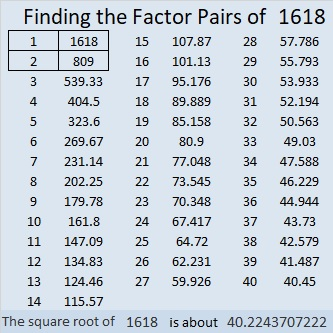# 1618 Math Happens in the Austin Chronicle

### Math Happens!

Several years before I started blogging, I tried to get my puzzles in newspapers, but the publishers of those newspapers just ignored them. Because of that, it is even sweeter to me that Math Happens put one of them in the Austin Chronicle! You can see it in the newspaper on page 25 of this pdf or in this cool page-turning e-edition. Math Happens in many different ways as you can see in their blog post from February 5. You can also look for Math Happens on a page in the middle of each of these  2020 issues or 2021 issues of the Austin Chronicle newspaper online.

Math Happens also in the Orange Leader, and they would love to also be in your local community newspaper.

### Today’s Puzzle:

Spring happens in just a few days! Today’s puzzle represents grasses blowing in a spring wind, readily anticipating the hiding of Easter eggs. It’s a level 3 puzzle, so start by finding the factors of the clue at the top of the puzzle (and the clue that goes with it), and work your way down cell by cell until you have written all the numbers from 1 to 12 in both the factor column and the factor row. You can do this!### Factors of 1618:

• 1618 is a composite number.
• Prime factorization: 1618 = 2 × 809.
• 1618 has no exponents greater than 1 in its prime factorization, so √1618 cannot be simplified.
• The exponents in the prime factorization are 1 and 1. Adding one to each exponent and multiplying we get (1 + 1)(1 + 1) = 2 × 2 = 4. Therefore 1618 has exactly 4 factors.
• The factors of 1618 are outlined with their factor pair partners in the graphic below.### More about the Number 1618:

1618 = 2 × 809, and 2809 is a perfect square. Thank you OEIS.org for that fun fact!

1618 is the sum of two squares:
33² + 23² = 1618.

1618 is the hypotenuse of a Pythagorean triple:
560-1518-1618, calculated from 2(33)(23), 33² – 23², 33² + 23².
It is also 2 times (280-759-809).

1618 is the 22nd centered heptagonal number because it is one more than seven times the 21st triangular number:
7(21)(22)/2 + 1 = 1618.

1618 has exactly four factors. The last number with exactly four factors was 1603. That’s the biggest gap so far between two numbers with exactly four factors!
(It will be interesting to see who will win the horse race for the current set of 100 numbers. So far, the horses for 2 factors and 8 factors are each running twice as fast as the horse for 4 factors, and 1619 will be a prime number, giving 2 factors the lead!)

A lot of math is happening with this number!